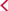返回

7速S tronic®双离合变速器
7速S tronic®双离合变速器
7速S tronic®双离合变速器
7速S tronic®双离合变速器
7速S tronic®双离合变速器
7速S tronic®双离合变速器
7速S tronic®双离合变速器
7速S tronic®双离合变速器

quattro四轮驱动
quattro四轮驱动

200
200
200
200
200
200
200
200

10.4
10.4
10.4
10.4
8.8
8.8
8.5
7.4

8.4
8.4
8.4
8.4
8.6
8.6
9.3
10.1

5.7
5.7
5.7
5.7
5.8
5.8
6.2
6.8

6.7
6.7
6.7
6.7
6.8
6.8
7.3
8.0

4481x1848x1616
4495x1848x1616
4481x1848x1616
4495x1848x1616
4481x1848x1616
4495x1848x1616
4495x1848x1616
4495x1848x1616

2680
2680
2680
2680
2680
2680
2680
2680

450
450
450
450
450
450
450
450

60
60
60
60
60
60
62.5
62.5

1570
1570
1570
1570
1625
1625
1725
1735

2065
2065
2065
2065
2110
2110
2210
2220

12
12
12
12
12
12
12
12

152
152
152
152
152
152
152
152

1395
1395
1395
1395
1984
1984
1984
1984

110/5000-6000
110/5000-6000
110/5000-6000
110/5000-6000
137/5000
137/5000
137/5000
162/4500-6200

250/1750-3000
250/1750-3000
250/1750-3000
250/1750-3000
320/1600-4000
320/1600-4000
320/1600-4000
350/1500-4400

LED前大灯
LED后尾灯带动态转向灯

-
-
-
-
-
-
-

-
-
-
-

-
-
-
-
-

-
-
-

-
-
-
-
-
-

-
-
-
-
-
-

-
-
-
-
-
-
-

-
-
-
-
-
-

-
-
-

-
-
-
-
-

-
-
-

-
-
-
-
-

-
-
-
-
-
-

-
-
-
-
-
-

-
-
-
-

-
-
-
-
-
-
-

-
-
-

-
-
-
-
-

-
-
-
3幅多功能真皮运动方向盘
-
-
-
-
3幅平底多功能真皮运动方向盘带换档拔片
-
-
-
-

-
-

-
-

-
-
-
-
-
-

-
5辐18英寸铝合金轮辋，7J x 18，轮胎 235/55 R18
o(￥0)
-
-
-
-
-
-
5双辐18英寸铝合金轮辋，7J x 18，轮胎 235/55 R18
o(￥0)
-
-
-
-
-
-
5双辐19英寸铝合金轮辋，7J x 19，轮胎 235/50 R19
o(￥2,500)
o(￥2,500)
o(￥0)
o(￥0)
o(￥0)
o(￥0)
5辐19英寸铝合金轮辋，7J x 19，轮胎 235/50 R19
o(￥2,500)
o(￥2,500)
o(￥0)
o(￥0)

o(￥2,500)
o(￥2,500)
o(￥2,500)
o(￥2,500)

-
-
o(￥6,000)
o(￥6,000)
o(￥6,000)
o(￥6,000)
o(￥6,000)

o(￥500)
o(￥500)
o(￥500)
o(￥500)
o(￥500)
o(￥500)
o(￥500)

o(￥0)
-
o(￥0)
-
o(￥0)
-
-
-

-
o(￥0)
-
o(￥0)
-
o(￥0)
o(￥0)
o(￥0)

o(￥1,000)
o(￥1,000)

o(￥2,000)
o(￥2,000)
o(￥1,000)
o(￥1,000)
o(￥1,000)
o(￥1,000)
o(￥1,000)
o(￥1,000)

-
-
o(￥800)
o(￥800)
o(￥800)
o(￥800)
o(￥800)
o(￥800)

-
o(￥0)
o(￥0)
o(￥0)
o(￥0)
o(￥0)

o(￥2,500)
o(￥2,500)
o(￥2,500)
o(￥2,500)
o(￥2,500)
o(￥2,500)
o(￥2,500)
3幅多功能带换档拨片真皮运动方向盘
o(￥1,000)
o(￥1,000)
o(￥1,000)
-
o(￥1,000)
-
-
-
3幅多功能带加热功能的真皮运动方向盘
o(￥1,000)
o(￥1,000)
o(￥1,000)
o(￥0)
o(￥1,000)
o(￥0)
o(￥0)
o(￥0)

o(￥3,500)
o(￥3,500)
-
-
Bang&Olufsen高级音响系统，带有3D音效
o(￥6,000)
o(￥6,000)
o(￥6,000)
o(￥6,000)
o(￥6,000)
o(￥6,000)
o(￥6,000)

o(￥2,000)
o(￥2,000)
o(￥2,000)
o(￥2,000)
o(￥2,000)
o(￥2,000)
o(￥2,000)
o(￥2,000)

-
-
o(￥13,000)
o(￥13,000)
o(￥13,000)
o(￥13,000)
o(￥13,000)

o(￥1,000)
o(￥1,000)
-

-
-
o(￥13,000)
o(￥13,000)
o(￥13,000)
o(￥13,000)
o(￥13,000)

-
-
o(￥3,500)
o(￥3,500)
o(￥3,500)
o(￥3,500)
o(￥3,500)
o(￥3,500)

-
-
o(￥5,500)
o(￥5,500)
o(￥5,500)
o(￥5,500)
o(￥5,500)
o(￥5,500)

-
-
o(￥9,000)
o(￥9,000)
o(￥9,000)
o(￥9,000)
o(￥9,000)
o(￥9,000)

-
-
o(￥1,500)
o(￥1,500)
o(￥1,500)
o(￥1,500)
o(￥1,500)
o(￥1,500)

o(￥1,500)
o(￥1,500)
o(￥1,500)
o(￥1,500)
o(￥1,500)
o(￥1,500)
o(￥1,500)

o(￥2,500)
o(￥2,500)
-

o(￥7,500)
o(￥7,500)
o(￥5,000)
o(￥5,000)
o(￥5,000)
o(￥5,000)
o(￥5,000)

o(￥2,000)
o(￥2,000)
o(￥2,000)
o(￥2,000)
o(￥2,000)
o(￥2,000)
o(￥2,000)

o(￥500)
o(￥500)
o(￥500)
o(￥500)
o(￥500)
o(￥500)
o(￥500)
o(￥500)

(1) ● 标准装备 ○可以选装 — 不提供 。
(2) 本目录内容根据印制时车辆配置状态信息编制。鉴于一汽-大众汽车有限公司不断对汽车进行改型和改进， 市场销售车型的部分配置及规格可能与本目录有所